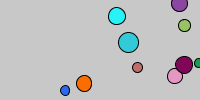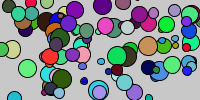# Bouncing Balls

example processing array animation random

Note: This example uses parallel arrays. In other words, we’re storing our data across multiple arrays. This is a good way to learn about arrays, but in real life you should use classes instead of parrallel arrays. If you haven’t learned about classes yet, don’t worry about it too much.

``````int ballCount = 10;

float[] x = new float[ballCount];
float[] y = new float[ballCount];
float[] xSpeed = new float[ballCount];
float[] ySpeed = new float[ballCount];
float[] size = new float[ballCount];
float[] r = new float[ballCount];
float[] g = new float[ballCount];
float[] b = new float[ballCount];

void setup() {
size(200, 100);
for(int i = 0; i < ballCount; i++){
x[i] = random(width);
y[i] = random(height);
xSpeed[i] = random(-5, 5);
ySpeed[i] = random(-5, 5);
size[i] = random(5, 20);
r[i] = random(256);
g[i] = random(256);
b[i] = random(256);
}
}

void draw() {

background(200);

for(int i = 0; i < ballCount; i++){

x[i] += xSpeed[i];
if(x[i] < 0 || x[i] > width){
xSpeed[i] *= -1;
}

y[i] += ySpeed[i];
if(y[i] < 0 || y[i] > height){
ySpeed[i] *= -1;
}

fill(r[i], g[i], b[i]);
ellipse(x[i], y[i], size[i], size[i]);

}
}
``````Code Editor

See the Pen by Happy Coding (@KevinWorkman) on CodePen.

Now that we have this code, we can easily make our program show `100` balls, just by changing the first line:

``````int ballCount = 100;
``````Or we could even make it show `1000` balls:

``````int ballCount = 1000;
``````